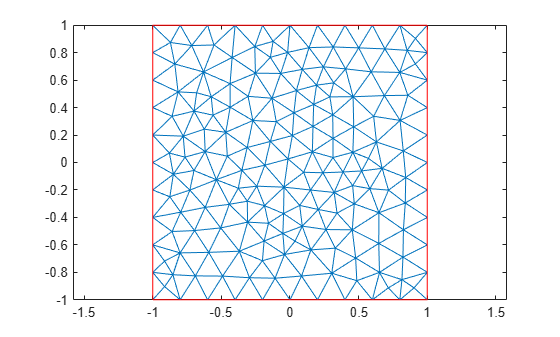pdetrg

(Not recommended) Triangle geometry data

pdetrg is not recommended. Use area instead.

Description

example

[ar,a1,a2,a3] = pdetrg(p,t) returns the areas of individual mesh triangles as a vector ar and half of the negative cotangent of each angle as vectors a1,a2,a3.

example

[ar,g1x,g1y,g2x,g2y,g3x,g3y] = pdetrg(p,t) returns the areas of individual mesh triangles as a vector ar and the gradient components of the triangle base functions as vectors g1x,g1y,g2x,g2y,g3x,g3y.

Examples

collapse all

Find the area of each element of a 2-D mesh. In addition, return half of the negative cotangent of each angle and the gradient components of the triangle base functions for each element.

Generate and plot a mesh for a unit square geometry.

[p,e,t] = initmesh(@squareg);
pdemesh(p,e,t)Compute the area of each individual element of the mesh and half of the negative cotangent of each angle. Display the first 5 elements for each result.

[ar,a1,a2,a3] = pdetrg(p,t);
ar(1:5)
ans = 1×5

0.0126    0.0148    0.0144    0.0156    0.0118

a1(1:5)
ans = 1×5

-0.2819   -0.3905   -0.5332   -0.1812   -0.5237

a2(1:5)
ans = 1×5

-0.5124   -0.2842   -0.1613   -0.4616   -0.3267

a3(1:5)
ans = 1×5

-0.1329   -0.2061   -0.2362   -0.2588   -0.0928

Find the area of the smallest and the largest element of the mesh.

min(ar)
ans = 0.0061
max(ar)
ans = 0.0216

Use the syntax with seven output arguments to compute the gradient components of the triangle base functions for each element.

[ar,g1x,g1y,g2x,g2y,g3x,g3y] = pdetrg(p,t);

Input Arguments

collapse all

Mesh nodes, specified as a 2-by-Np matrix of nodes (points), where Np is the number of nodes in the mesh. For details on the mesh data representation, see initmesh.

Data Types: double

Mesh elements, specified as a 4-by-Nt matrix of triangles, where Nt is the number of triangles in the mesh. For details on the mesh data representation, see initmesh.

Data Types: double

Output Arguments

collapse all

Areas of individual elements, returned as a row vector of positive numbers.

Half of the negative cotangent of each angle, returned as three row vectors of numbers.

Gradient components of the triangle base functions, returned as six row vectors of numbers.